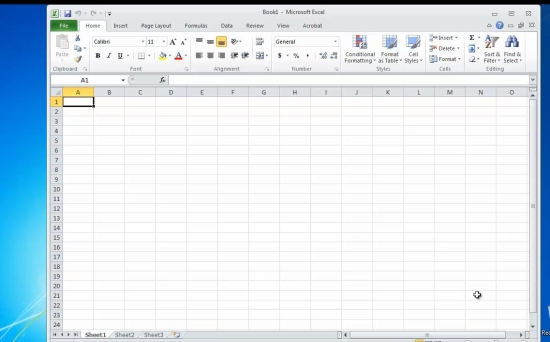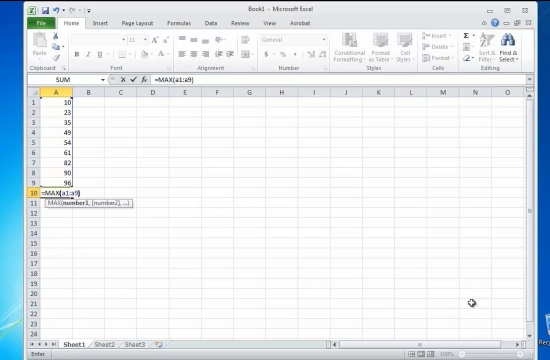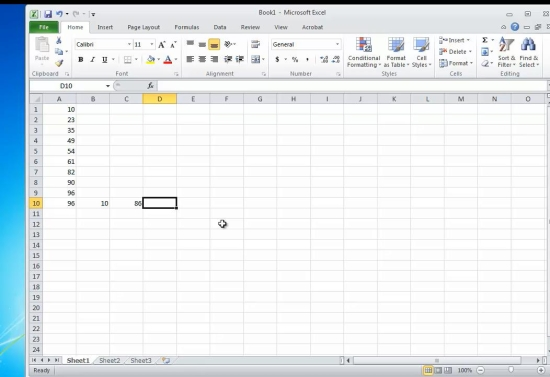## How to Calculate the Range in Excel

In this tutorial you will learn how to calculate range in Excel.

The range is the difference between the highest and the lowest of differences in a series of numbers.

We will therefore need the series that we want to perform range function upon.

Let’s create it:

Prerequisites: Microsoft Excel.

Step 1. Launch Excel and start a new file from File- New.Step 2. Fill several rows with the values you want to calculate the range for.

At the bottom of the column, type the following formula

=MAX (Axx:Ayy), where

Axx and Ayy are the starting, and the ending cells of your data, respectively.

Near the previous cell, type the following formula

=MIN(Axx:Ayy), where

Axx and Ayy are the starting, and the ending cells of your data, respectively.Step 3. Now go to the place where you want to have your range displayed.

Enter the formula

=X-Y, where X and Y are the first and the second cells calculated.

Hit Enter. Excel will update your cell with the result.Result: Congratulations, you have learnt how to calculate the range in Excel.